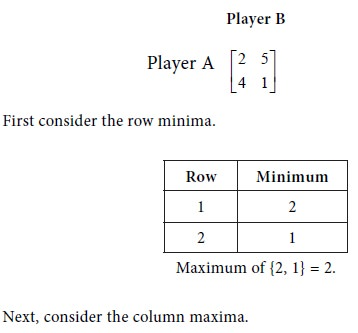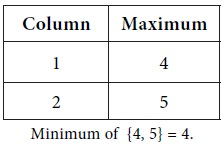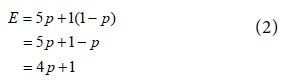Home | ARTS | Operations Management | Example of Graphical Solution Of A 2X2 Game With No Saddle Point

# Example of Graphical Solution Of A 2X2 Game With No Saddle Point

Posted On :  25.06.2018 12:14 am

Consider the game with the following pay-off matrix.

Example of Graphical Solution Of A 2X2 Game With No Saddle Point

Consider the game with the following pay-off matrix.We see that Maximum { row minima}  Minimum { column maxima }

So, the game has no saddle point. It is a mixed game.

Equations involving probability and expected value

Let p be the probability that player A will use his first strategy.

Then the probability that A will use his second strategy is 1-p.

Let E be the expected value of pay-off to player A.

When B uses his first strategy

The expected value of pay-off to player A is given byWhen B uses his second strategy

The expected value of pay-off to player A is given byConsider equations (1) and (2). For plotting the two equations on a graph sheet, get some points on them as follows:Tags : Operations Management - Game Theory, Goal Programming & Queuing Theory
Last 30 days 2123 views